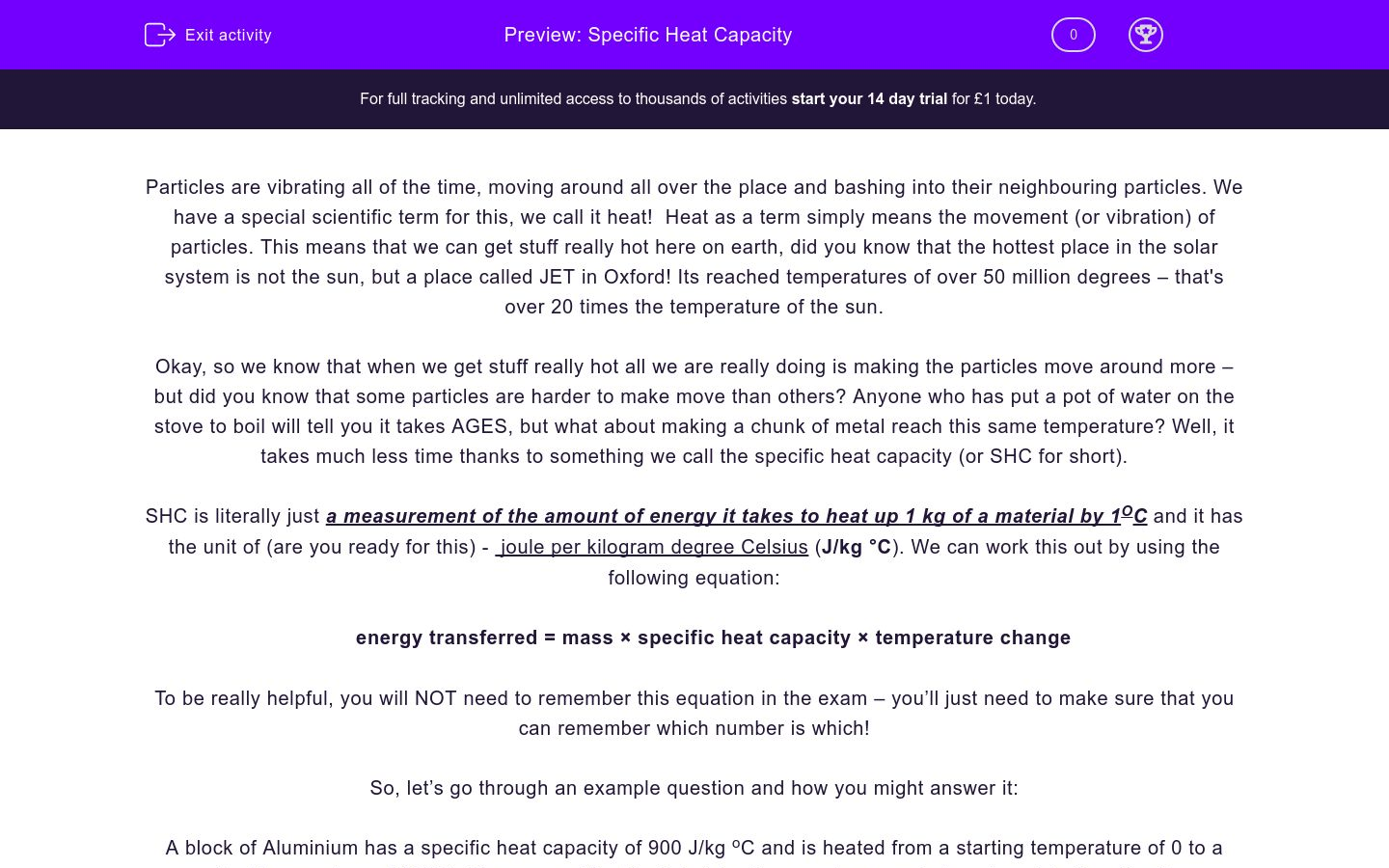# Energy Changes 1

In this worksheet, student learn how energy changes into different types and how to measure those changes.Key stage:  KS 4

Curriculum topic:  Physics: Energy

Curriculum subtopic:  Energy Changes

Difficulty level:### QUESTION 1 of 10

Hot objects have a high temperature, which means they have a lot of thermal energy (heat). The more heat an object has, the more quickly it loses it into the surroundings. The unit of temperature is °C (degrees Celsius) and that of heat energy is joule (J).

Ice cubes, for example, melt once they are out of the freezer. This is because they lose their heat energy to the surrounding environment. If you hold an ice cube, your hand gets colder, because heat from your hand goes to the ice cube. Remember: Thermal energy moves from hot to cold, causing the temperature of the hot object to decrease.

When thermal energy flows from object to object, we may have a change of state. The graph shows how temperature changes during changes of state:While a solid object is exposed to a higher temperature, its temperature increases and, when it reaches the melting point, it melts to become a liquid. While it melts, the temperature remains constant. The temperature then increases again until the boiling point is reached, when the temperature stabilises and the liquid now evaporates to become a gas.

The amount of energy needed to change the temperature of an object depends on its material. This property is called specific heat capacity. Its exact definition is: the amount of energy needed to change the temperature of one kilogram of a substance by one degree Celsius. The unit is joule per kilogram degree Celsius (J/kg °C). The formula is as follows:

energy transferred = mass × specific heat capacity × temperature change

The specific heat capacity of a material is a constant number, which will be given to you in an exam if you need it.

Another property substances have is specific latent heat. This is the energy needed to change the state of one kilogram of a substance without a change in temperature. Its unit is joule per kilogram (J/kg). Have a look at this formula:

energy transferred = mass × specific latent heat

The specific latent heat of a material is a constant number, which will be given to you in an exam if you need it.

What type of energy is heat?

kinetic

thermal

electrical

Complete the following sentence by selecting one of the options below:

Heat energy flows from...

cold to hot

hot to cold

Joule is the unit of measurement for......

temperature

specific latent heat

specific heat capacity

thermal energy

Is the following statement true or false?

While a substance is changing state the temperature remains constant.

true

false

In order for a substance to start melting, its temperature needs to reach its..........

boiling point

melting point

solidifying point

When the temperature of an object reaches its boiling point, it starts.......

melting

evaporating

solidifying

What is the unit of measurement for specific latent heat?

J/kg

kg/J

J/g

Mercury has a specific heat capacity of 140 J/kg °C. Calculate the energy transferred in kJ when 50kg of mercury are heated from 20°C to 25°C (just write the number in the box below).

Calculate the energy transferred in kJ when 2kg of water become gas at 100°C. The specific latent heat of water to steam is 2,260,000 J/kg.

What mass of ice needs 4 000 kJ to melt? Specific latent heat of ice to water is 340 000 J/kg.

HINT: 4 000 kJ = 4 000 000 J.

11.76 kg

11.86 kg

11.96 kg

• Question 1

What type of energy is heat?

thermal
EDDIE SAYS
Thermal energy is commonly called heat.
• Question 2

Complete the following sentence by selecting one of the options below:

Heat energy flows from...

hot to cold
EDDIE SAYS
Thermal energy flows from hot to cold.
• Question 3

Joule is the unit of measurement for......

thermal energy
EDDIE SAYS
Joule is the unit of measurement for thermal energy.
• Question 4

Is the following statement true or false?

While a substance is changing state the temperature remains constant.

true
EDDIE SAYS
While a substance is changing state the temperature remains constant. This is a true statement.
• Question 5

In order for a substance to start melting, its temperature needs to reach its..........

melting point
EDDIE SAYS
In order for a substance to start melting, its temperature needs to reach its melting point.
• Question 6

When the temperature of an object reaches its boiling point, it starts.......

evaporating
EDDIE SAYS
When the temperature of an object reaches its boiling point, it starts evaporating.
• Question 7

What is the unit of measurement for specific latent heat?

J/kg
EDDIE SAYS
The unit of measurement for specific latent heat is J/kg.
• Question 8

Mercury has a specific heat capacity of 140 J/kg °C. Calculate the energy transferred in kJ when 50kg of mercury are heated from 20°C to 25°C (just write the number in the box below).

35
EDDIE SAYS
Energy transferred = 50 x 140 x 5 = 35 000 J
1 kJ = 1000 J so 35 000 J =35 kJ
• Question 9

Calculate the energy transferred in kJ when 2kg of water become gas at 100°C. The specific latent heat of water to steam is 2,260,000 J/kg.

4520
4,520
EDDIE SAYS
Energy transferred = 2 x 2 260 000 = 4 520 000 J
= 4 520 kJ
• Question 10

What mass of ice needs 4 000 kJ to melt? Specific latent heat of ice to water is 340 000 J/kg.

HINT: 4 000 kJ = 4 000 000 J.